# Flow measurement

Flow measurement

Flow measurement is the quantification of bulk fluid movement. Flow can be measured in a variety of ways. Positive-displacement flow meters acumulate a fixed volume of fluid and then count the number of times the volume is filled to measure flow. Other flow measurement methods rely on forces produced by the flowing stream as it overcomes a known constriction, to indirectly calculate flow. Flow may be measured by measuring the velocity of fluid over a known area.

## Units of measurement

Both gas and liquid flow can be measured in volumetric or mass flow rates, such as litres per second or kilograms per second. These measurements can be converted between one another if the material's density is known. The density for a liquid is almost independent of the liquid conditions; however, this is not the case for gas, the density of which depends greatly upon pressure, temperature and to a lesser extent, the gas composition.

When gases or liquids are transferred for their energy content, such as the sale of natural gas, the flow rate may also be expressed in terms of energy flow, such as GJ/hour or BTU/day. The energy flow rate is the volume flow rate multiplied by the energy content per unit volume or mass flow rate multiplied by the energy content per unit mass. Where accurate energy comes to the time of the legit flow rate is desired, most flow meters will be used to calculate the volume or mass flow rate which is then adjusted to the energy flow rate by the use of a flow computer.

In engineering contexts, the volumetric flow rate is usually given the symbol Q, and the mass flow rate, the symbol$\dot m$.

### Gas

Gases are compressible and change volume when placed under pressure, are heated or are cooled. A volume of gas under one set of pressure and temperature conditions is not equivalent to the same gas under different conditions. References will be made to "actual" flow rate through a meter and "standard" or "base" flow rate through a meter with units such as acm/h (actual cubic meters per hour), kscm/h (kilo standard cubic meters per hour), LFM (linear feet per minute), or MSCFD (thousands of standard cubic feet per day).

Gas mass flow rate can be directly measured, independent of pressure and temperature effects, with thermal mass flow meters, Coriolis mass flow meters, or mass flow controllers.

### Liquid

For liquids, various units are used depending upon the application and industry, but might include gallons (U.S. liquid or imperial) per minute, liters per second, bushels per minute or, when describing river flows, cumecs (cubic metres per second) or acre-feet per day. In oceanography a common unit to measure volume transport (volume of water transported by a current for example) is a sverdrup (Sv) equivalent to 106 m3 / s.

## Mechanical flow meters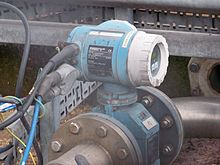A magnetic flow meter at the Tetley's Brewery in Leeds, West Yorkshire.

### Bucket-and-stopwatch

Perhaps the simplest way to measure volumetric flow is to measure how long it takes to fill a known volume container. A simple example is using a bucket of known volume, filled by a fluid. The stopwatch is started when the flow starts, and stopped when the bucket overflows. The volume divided by the time gives the flow. The bucket-and-stopwatch method is an off-line method, meaning that the measurement cannot be taken without interrupting the normal flow .

### Piston meter/Rotary piston

Because they are used for domestic water measurement, piston meters, also known as rotary piston or semi-positive displacement meters, are the most common flow measurement devices in the UK and are used for almost all meter sizes up to and including 40 mm (1½ʺ). The piston meter operates on the principle of a piston rotating within a chamber of known volume. For each rotation, an amount of water passes through the piston chamber. Through a gear mechanism and, sometimes, a magnetic drive, a needle dial and odometer type display are advanced.

### Variable area meter

The variable area (VA) meter, also commonly called a rotameter, consists of a tapered tube, typically made of glass, with a float inside that is pushed up by fluid flow and pulled down by gravity. As flow rate increases, greater viscous and pressure forces on the float cause it to rise until it becomes stationary at a location in the tube that is wide enough for the forces to balance. Floats are made in many different shapes, with spheres and spherical ellipses being the most common. Some are designed to spin visibly in the fluid stream to aid the user in determining whether the float is stuck or not. Rotameters are available for a wide range of liquids but are most commonly used with water or air. They can be made to reliably measure flow down to 1% accuracy.

### Turbine flow meter

The turbine flow meter (better described as an axial turbine) translates the mechanical action of the turbine rotating in the liquid flow around an axis into a user-readable rate of flow (gpm, lpm, etc.). The turbine tends to have all the flow traveling around it.

The turbine wheel is set in the path of a fluid stream. The flowing fluid impinges on the turbine blades, imparting a force to the blade surface and setting the rotor in motion. When a steady rotation speed has been reached, the speed is proportional to fluid velocity.

Turbine flow meters are used for the measurement of natural gas and liquid flow. Turbine meters are less accurate than displacement and jet meters at low flow rates, but the measuring element does not occupy or severely restrict the entire path of flow. The flow direction is generally straight through the meter, allowing for higher flow rates and less pressure loss than displacement-type meters. They are the meter of choice for large commercial users, fire protection, and as master meters for the water distribution system. Strainers are generally required to be installed in front of the meter to protect the measuring element from gravel or other debris that could enter the water distribution system. Turbine meters are generally available for 1-1/2" to 12" or higher pipe sizes. Turbine meter bodies are commonly made of bronze, cast Iron, or ductile iron. Internal turbine elements can be plastic or non-corrosive metal alloys. they are accurate in normal working conditions to 0.2l/s however are affect greatly with dog mix interference.

Fire meters are a specialized type of turbine meter with approvals for the high flow rates required in fire protection. They are often approved by Underwriters Laboratories (UL) or Factory Mutual (FM) for use in fire protection.

Fire hydrant meters are a specialized type of portable turbine meter that are attached to a fire hydrant to measure water out of the hydrant. The meters are normally made of aluminum to be light weight, and are usually 3" capacity. Utilities often require them for measurement of water used in construction, pool filling, or where a permanent meter is not yet installed.

### Woltmann meter

The Woltmann meter comprises a rotor with helical blades inserted axially in the flow, much like a ducted fan; it can be considered a type of turbine flow meter. They are commonly referred to as helix meters, and are popular at larger sizes.

### Single jet meter

A single jet meter consists of a simple impeller with radial vanes, impinged upon by a single jet. They are increasing in popularity in the UK at larger sizes and are commonplace in the EU.

This is similar to the single jet meter, except that the impeller is small with respect to the width of the pipe, and projects only partially into the flow, like the paddle wheel on a Mississippi riverboat.

### Multiple jet meter

A multiple jet or multijet meter is a velocity type meter which has an impeller which rotates horizontally on a vertical shaft. The impeller element is in a housing in which multiple inlet ports direct the fluid flow at the impeller causing it to rotate in a specific direction in proportion to the flow velocity. This meter works mechanically much like a single jet meter except that the ports direct the flow at the impeller equally from several points around the circumference of the element, not just one point; this minimizes uneven wear on the impeller and its shaft.

### Pelton wheel

The Pelton wheel turbine (better described as a radial turbine) translates the mechanical action of the Pelton wheel rotating in the liquid flow around an axis into a user-readable rate of flow (gpm, lpm, etc.). The Pelton wheel tends to have all the flow traveling around it with the inlet flow focused on the blades by a jet. The original Pelton wheels were used for the generation of power and consisted of a radial flow turbine with "reaction cups" which not only move with the force of the water on the face but return the flow in opposite direction using this change of fluid direction to further increase the efficiency of the turbine.

### Oval gear meter

An oval gear meter is a positive displacement meter that uses two or more oblong gears configured to rotate at right angles to one another, forming a T shape. Such a meter has two sides, which can be called A and B. No fluid passes through the center of the meter, where the teeth of the two gears always mesh. On one side of the meter (A), the teeth of the gears close off the fluid flow because the elongated gear on side A is protruding into the measurement chamber, while on the other side of the meter (B), a cavity holds a fixed volume of fluid in a measurement chamber. As the fluid pushes the gears, it rotates them, allowing the fluid in the measurement chamber on side B to be released into the outlet port. Meanwhile, fluid entering the inlet port will be driven into the measurement chamber of side A, which is now open. The teeth on side B will now close off the fluid from entering side B. This cycle continues as the gears rotate and fluid is metered through alternating measurement chambers. Permanent magnets in the rotating gears can transmit a signal to an electric reed switch or current transducer for flow measurement.

### Nutating disk meter

This is the most commonly used measurement system for measuring water supply. The fluid, most commonly water, enters in one side of the meter and strikes the nutating disk, which is eccentrically mounted. The disk must then "wobble" or nutate about the vertical axis, since the bottom and the top of the disk remain in contact with the mounting chamber. A partition separates the inlet and outlet chambers. As the disk nutates, it gives direct indication of the volume of the liquid that has passed through the meter as volumetric flow is indicated by a gearing and register arrangement, which is connected to the disk. It is reliable for flow measurements within 1 percent.

### Current meter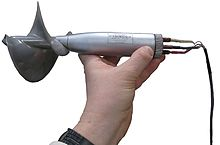A propeller-type current meter as used for hydroelectric turbine testing.

Flow through a large penstock such as used at a hydroelectric power plant can be measured by averaging the flow velocity over the entire area. Propeller-type current meters (similar to the purely mechanical Ekman current meter, but now with electronic data acquisition) can be traversed over the area of the penstock and velocities averaged to calculate total flow. This may be on the order of hundreds of cubic meters per second. The flow must be kept steady during the traverse of the current meters. Methods for testing hydroelectric turbines are given in IEC standard 41. Such flow measurements are often commercially important when testing the efficiency of large turbines.

## Pressure-based meters

There are several types of flow meter that rely on Bernoulli's principle, either by measuring the differential pressure within a constriction, or by measuring static and stagnation pressures to derive the dynamic pressure.

### Venturi meter

A Venturi meter constricts the flow in some fashion, and pressure sensors measure the differential pressure before and within the constriction. This method is widely used to measure flow rate in the transmission of gas through pipelines, and has been used since Roman Empire times.The coefficient of discharge of Venturi meter ranges from 0.93 to 0.97.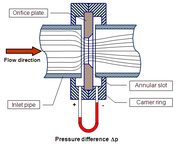ISO 5167 Orifice Plate

### Orifice plate

An orifice plate is a plate with a hole through it, placed in the flow; it constricts the flow, and measuring the pressure differential across the constriction gives the flow rate. It is basically a crude form of Venturi meter, but with higher energy losses. There are three type of orifice: concentric, eccentric, and segmental.

### Dall tube

The Dall tube is a shortened version of a Venturi meter, with a lower pressure drop than an orifice plate. As with these flow meters the flow rate in a Dall tube is determined by measuring the pressure drop caused by restriction in the conduit. The pressure differential is typically measured using diaphragm pressure transducers with digital readout. Since these meters have significantly lower permanent pressure losses than orifice meters, Dall tubes are widely used for measuring the flow rate of large pipeworks.

### Pitot tube

A Pitot tube is a pressure measuring instrument used to measure fluid flow velocity by determining the stagnation pressure. Bernoulli's equation is used to calculate the dynamic pressure and hence fluid velocity.

### Multi-hole pressure probe

Multi-hole pressure probes (also called impact probes) extend the theory of pitot tube to more than one dimension. A typical impact probe consists of three or more holes (depending on the type of probe) on the measuring tip arranged in a specific pattern. More holes allow the instrument to measure the direction of the flow velocity in addition to its magnitude (after appropriate calibration). Three holes arranged in a line allow the pressure probes to measure the velocity vector in two dimensions. Introduction of more holes, e.g. five holes arranged in a "plus" formation, allow measurement of the three-dimensional velocity vector.

## Optical flow meters

Optical flow meters use light to determine flow rate. Small particles which accompany natural and industrial gases pass through two laser beams focused a short distance apart in the flow path. in a pipe by illuminating optics. Laser light is scattered when a particle crosses the first beam. The detecting optics collects scattered light on a photodetector, which then generates a pulse signal. As the same particle crosses the second beam, the detecting optics collect scattered light on a second photodetector, which converts the incoming light into a second electrical pulse. By measuring the time interval between these pulses, the gas velocity is calculated as V = D / t where D is the distance between the laser beams and t is the time interval.

Laser-based optical flow meters measure the actual speed of particles, a property which is not dependent on thermal conductivity of gases, variations in gas flow or composition of gases. The operating principle enables optical laser technology to deliver highly accurate flow data, even in challenging environments which may include high temperature, low flow rates, high pressure, high humidity, pipe vibration and acoustic noise.

Optical flow meters are very stable with no moving parts and deliver a highly repeatable measurement over the life of the product. Because distance between the two laser sheets does not change, optical flow meters do not require periodic calibration after their initial commissioning. Optical flow meters require only one installation point, instead of the two installation points typically required by other types of meters. A single installation point is simpler, requires less maintenance and is less prone to errors.

Commercially available ptical flow meters are capable of measuring flow from 0.1 m/s to faster than 100 m/s (1000:1 turn down ratio) and have been demonstrated to be effective for the measurement of flare gases from oil wells and refineries, a contributor to atmospheric pollution.

## Open channel flow measurement

### Level to flow

The level of the water is measured at a designated point behind a hydraulic structure (a weir or flume) using various means (bubblers, ultrasonic, float, and differential pressure are common methods). This depth is converted to a flow rate according to a theoretical formula of the form Q = KHX where Q is the flow rate, K is a constant, H is the water level, and X is an exponent which varies with the device used; or it is converted according to empirically derived level/flow data points (a "flow curve"). The flow rate can then integrated over time into volumetric flow.

### Area / velocity

The cross-sectional area of the flow is calculated from a depth measurement and the average velocity of the flow is measured directly (Doppler and propeller methods are common). Velocity times the cross-sectional area yields a flow rate which can be integrated into volumetric flow.

### Dye testing

A known amount of dye (or salt) per unit time is added to a flow stream. After complete mixing, the concentration is measured. The dilution rate equals the flow rates.

### Acoustic Doppler velocimetry

Acoustic Doppler velocimetry (ADV) is designed to record instantaneous velocity components at a single point with a relatively high frequency. Measurements are performed by measuring the velocity of particles in a remote sampling volume based upon the Doppler shift effect.

## Thermal mass flow meters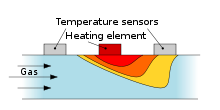Temperature at the sensors varies depending upon the mass flow

Thermal mass flow meters generally use combinations of heated elements and temperature sensors to measure the difference between static and flowing heat transfer to a fluid and infer its flow with a knowledge of the fluid's specific heat and density. The fluid temperature is also measured and compensated for. If the density and specific heat characteristics of the fluid are constant, the meter can provide a direct mass flow readout, and does not need any additional pressure temperature compensation over their specified range.

Technological progress has allowed the manufacture of thermal mass flow meters on a microscopic scale as MEMS sensors; these flow devices can be used to measure flow rates in the range of nanolitres or microlitres per minute.

Thermal mass flow meter technology is used for compressed air, nitrogen, helium, argon, oxygen, and natural gas. In fact, most gases can be measured as long as they are fairly clean and non-corrosive. For more aggressive gases, the meter may be made out of special alloys (e.g. Hastelloy), and pre-drying the gas also helps to minimize corrosion.

Today, thermal mass flow meters are used to measure the flow of gases in a growing range of applications, such as chemical reactions or thermal transfer applications that are difficult for other flow metering technologies. This is because thermal mass flow meters monitor variations in one or more of the thermal characteristics (temperature, thermal conductivity, and/or specific heat) of gaseous media to define the mass flow rate.

### The MAF sensor

In many late model automobiles, a mass airflow sensor (MAF sensor) is used to accurately determine the mass flowrate of intake air used in the internal combustion engine. Many such mass flow sensors utilize a heated element and a downstream temperature sensor to indicate the air flowrate. Other sensors use a spring-loaded vane. In either case, the vehicle's electronic control unit interprets the sensor signals as a real time indication of an engine's fuel requirement.

## Vortex flowmeters

Another method of flow measurement involves placing a bluff body (called a shedder bar) in the path of the fluid. As the fluid passes this bar, disturbances in the flow called vortices are created. The vortices trail behind the cylinder, alternatively from each side of the bluff body. This vortex trail is called the Von Kármán vortex street after von Kármán's 1912 mathematical description of the phenomenon. The frequency at which these vortices alternate sides is essentially proportional to the flow rate of the fluid. Inside, atop, or downstream of the shedder bar is a sensor for measuring the frequency of the vortex shedding. This sensor is often a piezoelectric crystal, which produces a small, but measurable, voltage pulse every time a vortex is created. Since the frequency of such a voltage pulse is also proportional to the fluid velocity, a volumetric flow rate is calculated using the cross sectional area of the flow meter. The frequency is measured and the flow rate is calculated by the flowmeter electronics using the equation f = SV / L where f is the frequency of the vortices, L the characteristic length of the bluff body, V is the velocity of the flow over the bluff body, and S is the Strouhal number, which is essentially a constant for a given body shape within its operating limits.

## Electromagnetic, ultrasonic and coriolis flow meters

Modern innovations in the measurement of flow rate incorporate electronic devices that can correct for varying pressure and temperature (i.e. density) conditions, non-linearities, and for the characteristics of the fluid.

### Magnetic flow meters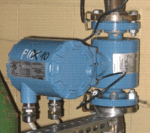Industrial magnetic flowmeter

The most common flow meter apart from mechanical flow meters is the magnetic flow meter, commonly referred to as a "mag meter" or an "electromag". A magnetic field is applied to the metering tube, which results in a potential difference proportional to the flow velocity perpendicular to the flux lines. The physical principle at work is Faraday's law of electromagnetic induction. The magnetic flow meter requires a conducting fluid, e.g. water, and an electrical insulating pipe surface, e.g. a rubber lined nonmagnetic steel tube.

### Ultrasonic (Doppler, transit time) flow meters

Ultrasonic flow meters measure the difference of the transit time of ultrasonic pulses propagating in and against flow direction. This time difference is a measure for the average velocity of the fluid along the path of the ultrasonic beam. By using the absolute transit times both the averaged fluid velocity and the speed of sound can be calculated. Using the two transit times tup and tdown and the distance between receiving and transmitting transducers L and the inclination angle α one can write the equations:$v = \frac{L}{{2\;\sin \left( \alpha \right)}}\;\frac{{t_{up} - t_{down} }}{{t_{up} \;t_{down} }}$ and$c = \frac{L}{2}\;\frac{{t_{up} + t_{down} }}{{t_{up} \;t_{down} }}$

where v is the average velocity of the fluid along the sound path and c is the speed of sound.

Ultrasonic flow meters are also used for the measurement of natural gas flow. One can also calculate the expected speed of sound for a given sample of gas; this can be compared to the speed of sound empirically measured by an ultrasonic flow meter and for the purposes of monitoring the quality of the flow meter's measurements. A drop in quality is an indication that the meter needs servicing. Recently, Ultrasonic flow meters are also being used for measurement of LNG flow.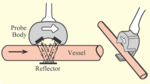Schematic view of a flow sensor.

Measurement of the Doppler shift resulting in reflecting an ultrasonic beam off the flowing fluid has also been used in the past. Due to limited accuracy this method is only suitable for applications that do not require a high accuracy. By passing an ultrasonic beam through the tissues, bouncing it off a reflective plate, then reversing the direction of the beam and repeating the measurement, the volume of blood flow can be estimated. The frequency of the transmitted beam is affected by the movement of blood in the vessel and by comparing the frequency of the upstream beam versus downstream the flow of blood through the vessel can be measured. The difference between the two frequencies is a measure of true volume flow. A wide-beam sensor can also be used to measure flow independent of the cross-sectional area of the blood vessel.

For the Doppler principle to work in a flowmeter it is mandatory that the flow stream contains sonically reflective materials, such as solid particles or entrained air bubbles.

A related technology is acoustic Doppler velocimetry.

### Coriolis flow meters

Using the Coriolis effect that causes a laterally vibrating tube to distort, a direct measurement of mass flow can be obtained in a coriolis flow meter. Furthermore a direct measure of the density of the fluid is obtained. Coriolis measurement can be very accurate irrespective of the type of gas or liquid that is measured; the same measurement tube can be used for hydrogen gas and bitumen without recalibration.

Coriolis flow meters can be used for the measurement of natural gas flow.

## Laser Doppler flow measurement

A beam of laser light impinging on a moving particle will be partially scattered with a change in wavelength proportional to the particle's speed (the Doppler effect). A Laser Doppler velocimeter (LDV), also called a laser Doppler anemometer (LDA), focuses a laser beam into a small volume in a flowing fluid containing small particles (naturally occurring or induced). The particles scatter the light with a Doppler shift. Analysis of this shifted wavelength can be used to directly, and with great precision, determine the speed of the particle and thus a close approximation of the fluid velocity.

A number of different techniques and device configurations are available for determining the Doppler shift. All use a photodetector (typically an avalanche photodiode) to convert the light into an electrical waveform for analysis. In most devices, the original laser light is divided into two beams. In one general LDV class, the two beams are made to intersect at their focal points where they interfere and generate a set of straight fringes. The sensor is then aligned to the flow such that the fringes are perpendicular to the flow direction. As particles pass through the fringes, the Doppler-shifted light is collected into the photodetector. In another general LDV class, one beam is used as a reference and the other is Doppler-scattered. Both beams are then collected onto the photodetector where optical heterodyne detection is used to extract the Doppler signal.

## Calibration

Even though ideally the flowmeter should be unaffected by its environment, in practice this is unlikely to be the case. Often measurement errors originate from incorrect installation or other environment dependent factors. In situ methods are used when flow meter is calibrated in the correct flow conditions.

### Transit time method

For pipe flows a so-called transit time method is applied where a radiotracer is injected as a pulse into the measured flow. The transit time is defined with the help of radiation detectors placed on the outside of the pipe. The volume flow is obtained by multiplying the measured average fluid flow velocity by the inner pipe cross section. This reference flow value is compared with the simultaneous flow value given by the flow measurement to be calibrated.

The procedure is standardised (ISO 2975/VII for liquids and BS 5857-2.4 for gases). The best accredited measurement uncertainty for liquids and gases is 0.5 %.

### Tracer dilution method

The radiotracer dilution method is used to calibrate open channel flow measurements. A solution with a known tracer concentration is injected at a constant known velocity into the channel flow. Downstream where the tracer solution is thoroughly mixed over the flow cross section, a continuous sample is taken and its tracer concentration in relation to that of the injected solution is determined. The flow reference value is determined by using the tracer balance condition between the injected tracer flow and the diluting flow..

The procedure is standardised (ISO 9555-1 and ISO 9555-2 for liquid flow in open channels). The best accredited measurement uncertainty is 1 %.

Wikimedia Foundation. 2010.

### Look at other dictionaries:

• Measurement Systems division — Measurement Systems (formerly NuFlo Measurement Systems), a division of Cameron, designs, manufactures and distributes measurement, quality, and control instrumentation for the global oil gas and process control industries. Contents 1 History 2… …   Wikipedia

• Measurement Science and Technology —   Abbreviated title (ISO …   Wikipedia

• Flow sensor — A flow sensor is a device for sensing the rate of fluid flow. Typically a flow sensor is the sensing element used in a flow meter, or flow logger, to record the flow of fluids.As is true for all sensors, absolute accuracy of a measurement… …   Wikipedia

• Flow cytometry — Analysis of a marine sample of photosynthetic picoplankton by flow cytometry showing three different populations (Prochlorococcus, Synechococcus, and picoeukaryotes) Flow cytometry (abbreviated: FCM) is a technique for counting and examining… …   Wikipedia

• Measurement while drilling — Well logging Gamma ray logging Spontaneous potential logging Resistivity logging Density logging Sonic logging Caliper logging Mud logging LWD/MWD v · …   Wikipedia

• measurement — /mezh euhr meuhnt/, n. 1. the act of measuring. 2. a measured dimension. 3. extent, size, etc., ascertained by measuring. 4. a system of measuring or measures: liquid measurement. [1745 55; MEASURE + MENT] * * * Association of numbers with… …   Universalium

• Flow computer — A flow computer is an electronic computational device which implements the required algorithms to turn the raw data received from flow meters to which it is connected into volumes at base conditions.A flow computer also audits changes that have… …   Wikipedia

• flow — 1. To bleed from the uterus less profusely than in flooding. 2. The menstrual discharge. 3. Movement of a liquid or gas; specifically, the volume of liquid or gas passing a given point per unit of …   Medical dictionary

• Measurement systems employed in petroleum refining — ▪ Table Measurement systems employed in petroleum refining                       units of measure refinery operation international U.S. quantity processed metric tons barrels (42 gallons per barrel) unit capacity tons per year barrels per day… …   Universalium

• Ultrasonic flow meter — A ultrasonic flow meter measures the velocity of a liquid or gas through a pipe using acoustic sensors. This has some advantages over other measurement techniques. The results are slightly affected by temperature, density or conductivity.… …   Wikipedia